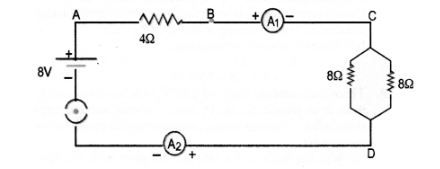# Find out the following in the circuit given in the figure.

Question:

Find out the following in the circuit given in the figure.(a) Effective resistance of two 8Ω resistors in the combination.

(b) Current flowing through 40 resistor

(c) Potentional difference across 40 resistor

(d) Power dissipated in 4Ω resistor

(e) Difference in ammeter readings, if any.

(CBSE 2010,2012)

Solution:

(a) Two 80 resistors are in parallel, so their effective resistance is given by

$\frac{1}{\mathrm{R}}=\frac{1}{8}+\frac{1}{8}=\frac{1}{4}$ or $\mathrm{R}=4 \Omega$

(b) Net resistance of the circuit, $\mathrm{R}=4 \Omega+4 \Omega=8 \Omega$

$V=8 V$

$\therefore$ Current in the ciruit, $\mathrm{I}=\frac{\mathrm{V}}{\mathrm{R}}=\frac{8}{8}=1 \mathrm{~A}$

Hence current flowing through $4 \Omega$ resistor $=1 \mathrm{~A}$

(c) Potential difference across $4 \Omega$ resistor, $\mathrm{V}=\mathrm{IR}$

$=1 \times 4=4 \mathrm{~V}$

(d) Power dissipated in $4 \Omega$ resistor, $\mathrm{P}=\mathrm{I}^{2} \mathrm{R}$

$=1 \times 4=4 W$

(e) Since same current flows through every part in a series circuit and both the ammeters are connected in series, so there will be no difference in ammeter readings.